# Angular Impulse Momentum Relations Civil Engineering (CE) Notes | EduRev

## Civil Engineering (CE) : Angular Impulse Momentum Relations Civil Engineering (CE) Notes | EduRev

The document Angular Impulse Momentum Relations Civil Engineering (CE) Notes | EduRev is a part of the Civil Engineering (CE) Course Introduction to Dynamics and Vibrations- Notes, Videos, MCQs.
All you need of Civil Engineering (CE) at this link: Civil Engineering (CE)

4.3.1 Definition of the angular impulse of a force

The angular impulse of a force is the time integral of the moment exerted by the force.

To make the concept precise, consider a particle that is subjected to a time varying force F(t) , with components in a fixed basis {i,j,k} , then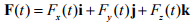Let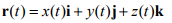denote the position vector of the particle, and

M(t)=r(t)xF(t) = (y(t)Fz(t)-z(t)Fy(t))i+(z(t)Fx(t)-x(t)Fz(t))j+(x(t)Fy(t)-y(t)Fz(t))k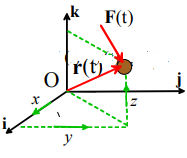the moment of the force about the origin.

The Angular Impulse exerted by the force about O during a time interval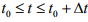is defined as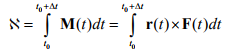The angular impulse is a vector, and can be expressed as components in a basis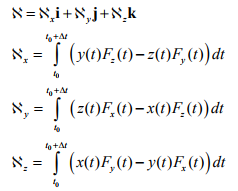If you know the moment as a function of time, you can calculate its angular impulse using simple calculus. For example for a constant moment, with vector value M0 , the impulse is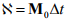4.3.2 Definition of the angular momentum of a particle

The angular momentum of a particle is simply the cross product of the particle’s position vector with its linear momentum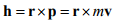The angular momentum is a vector – the direction of the vector is perpendicular to its velocity and its position vectors.

4.3.3 Angular impulse – Angular Momentum relation for a single particle

Consider a particle that is subjected to a force F(t) for a time interval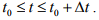Let r(t) denote the position vector of the particle

Let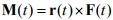denote the moment of F about the origin

Let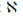denote the angular impulse exerted on the particle

Let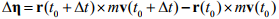denote the change in angular momentum during the time interval Δt.

The momentum conservation equation can be expressed either in differential or integral form.

1. In differential form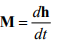2. In integral form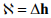Although it’s not obvious, these are just another way of writing Newton’s laws of motion. To show this, we’ll derive the differential form. Start with Newton’s law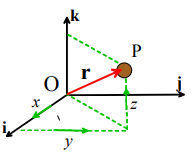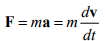Take the cross product of both sides with r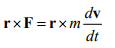Note that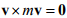since the cross product of two parallel vectors is zero. We can add this to the right hand side, which shows that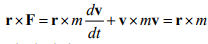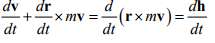This yields the required relation.

Angular momentum conservation: For the special case where the force is parallel to r, the moment of the force acting on the particle is zero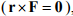and angular momentum is constant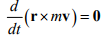4.3.4 Examples using Angular Impulse – Angular Momentum relations for a single particle

The angular impulse-angular momentum equations are particularly helpful when you need to solve problems where particles are subjected to a single force, which acts through a fixed point. They can also be used to analyze rotational motion of a massless frame containing one or more particles.

Example 1: Orbital motion. A satellite is launched into a geostationary transfer orbit by the ARIANE V launch facility. At its perigee (the point where the satellite is closest to the earth) the satellite has speed 10.2km/sec and altitude 250km. At apogee (the point where the satellite is furthest from the earth) the satellite has altitude 35950 km. Calculate the speed of the satellite at apogee.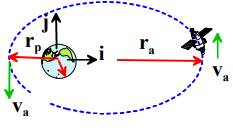Assumptions:

1. We assume that the only force acting on the satellite is the gravitational attraction of the earth
2. The earth’s radius is 6378.145km

Calculation:

1. Since the gravitational force on the satellite always acts towards the center of the earth, angular momentum about the earth’s center is conserved.
2. At both perigee and apogee, the velocity vector of the satellite must be perpendicular to its position vector. To see this, note that at the point where the satellite is closest and furthest from the earth, the distance to the earth is a max or min, and so the derivative of the distance of the satellite from the earth must vanish, i.e.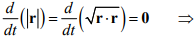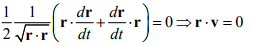where we have used the chain rule to evaluate the time derivative of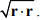Recall that if the dot product of two vectors vanishes, they are mutually perpendicular. We take a coordinate system with i and j in the plane of the orbit, and k perpendicular to the orbit.

3. We take the satellite orbit to lie in the i , j plane with k perpendicular to the orbit. The angular momentum at apogee and perigee is then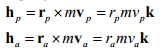where ra , rp are the distance of the satellite from the center of the earth at apogee and perigee, and va , vp are the corresponding speeds.

4. Since angular momentum is conserved it follows that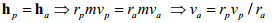5. Substituting numbers yields 1.6 km/s

Example 2: More orbital motion. The orbit for a satellite is normally specified by a set of angles specifying the inclination of the orbit, and by quoting the distance of the satellite from the earths center at apogee and perigee ra , rp It is possible to calculate the speed of the satellite at perigee and apogee from this information.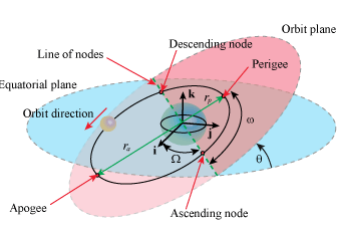Calculation

1. From the previous example, we know that the distances and velocities are related by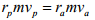2. The system is conservative, so the total energy of the system is conserved.

3. The potential energies when the satellite is at perigee and apogee are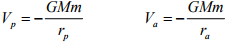4. The kinetic energies of the satellite at perigee and apogee are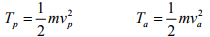5. The kinetic energy of the earth can be assumed to be constant. Energy conservation therefore shows that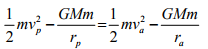6. The results of (1) and (5) give two equations that can be solved for va , vin terms of known parameters. For example, (1) shows that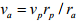- this can be substituted into (5) to see that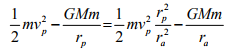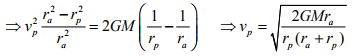Similarly, at apogee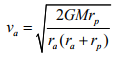Offer running on EduRev: Apply code STAYHOME200 to get INR 200 off on our premium plan EduRev Infinity!

## Introduction to Dynamics and Vibrations- Notes, Videos, MCQs

20 videos|53 docs

,

,

,

,

,

,

,

,

,

,

,

,

,

,

,

,

,

,

,

,

,

;